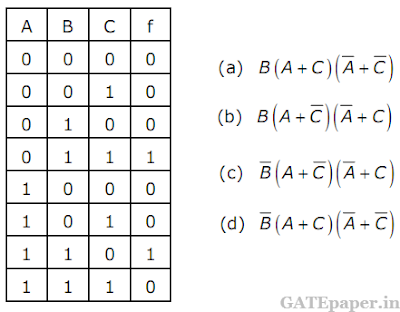### GATE 2005 ECE Video Solution on Digital Circuits (Digital Electronics)

1. Decimal 43 in Hexadecimal and BCD number system is respectively
a. B2, 0100 0011
b. 2B, 0100 0011
c. 2B, 0011 0100
d. B2, 0100 0100

2. The Boolean function f implemented in the figure using two input multiplexers is3. The Boolean expression for the truth table shown is4. The present output Qn of an edge triggered JK flip-flop is logic ‘0’. If j = 1, then Qn+1 is
a. Cannot be determined
b. Will be logic ‘0’
c. Will be logic ‘1’
d. Will race around

5. The given figure shows a ripple counter using positive edge triggered flip-flops. If the present state of the counter is Q2Q1Q0 = 011, then its next state Q2Q1Q0 will bea. 010
b. 100
c. 111
d. 101

6. What memory address range is NOT represented by chip #1 and chip #2 in the figure. A0 to A15 in this figure are the address lines and CS means chip select.a. 0100 – 02FF
b. 1500 – 16FF
c. F900 – FAFF
d. F800 – F9FF

Consider an 8085 microprocessor system.
7. The following program starts at location 0100H.
LXI SP, 00FF
LXI H, 0701
MVI A, 20H
SUB M
THE Contents of accumulator when the program counter reaches 0109H is
a. 20H
b. 02H
c. 00H
d. FFH

8. If in addition, following code exists from 0109H onwards.
ORI 40H
What will be the result in the accumulator after executing the last instruction?
a. 40H
b. 20H
c. 60H
d. 42H

Solution (7 & 8):

#### 1 comment:

1.Name

Email *

Message *